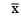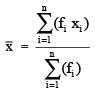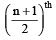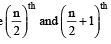Courses

# Facts that Matter- Statistics Class 9 Notes | EduRev

## Class 9 Mathematics by Full Circle

Created by: Full Circle

## Class 9 : Facts that Matter- Statistics Class 9 Notes | EduRev

The document Facts that Matter- Statistics Class 9 Notes | EduRev is a part of the Class 9 Course Class 9 Mathematics by Full Circle.
All you need of Class 9 at this link: Class 9

Facts that Matter

• Three measures of central tendency of ungrouped data are: (i) mean, (ii) median and (iii) mode.
• The mean of ungrouped data is found by adding all the values of observation and dividing it by the total number of observations. It is denoted by.• The median is the value of the middlemost observation.
• The mode is the most frequently occurring observation.
• If the number of observations ‘n’ is odd, then
Median = Value of theobservation.
• If the number of observations ‘n’ is even, then
Median = Mean value of the andobservations.

STATISTICS
It is a branch of science which deals with the collection, presentation, analysis and interpretation of numerical data.

DATA
Facts or numerical figures, collected with a definite purpose, are called data.
Ungrouped or raw data
The data obtained in original form are called raw data or ungrouped data.
Grouped data
The data condensed into groups or classes are called grouped data.

Note:
I. The arrangement of raw numerical data in ascending or descending order of magnitude, is called an array.
II. The number of times an observation occurs in the given data is called the frequency of that observation.

132 docs

,

,

,

,

,

,

,

,

,

,

,

,

,

,

,

,

,

,

,

,

,

;